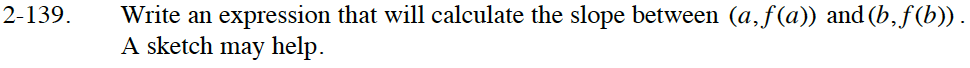### Home > CALC > Chapter 2 > Lesson 2.4.1 > Problem2-139

2-139.

Write an expression that will calculate the slope between (a, f(a)) and (b, f(b)). A sketch may help. Homework Help ✎$\text{slope }=\frac{\Delta y}{\Delta x}$